## 设置模块参数值

• 通过创建变量在模块和模型之间共享参数值。

• 通过创建参数对象来控制参数特征，如数据类型和维度。

• 使用数学表达式以代码的形式为算法建模。

### 以编程方式访问参数值

• 以编程方式构建模型。

• 以编程方式进行模型仿真时，在仿真运行期间调整参数值。

`paramValue = get_param('myModel/My Constant','Value')`
```paramValue = 15```

`set_param('myModel/My Constant','Value','25')`

### 指定参数值

`2.3`

`[1.2 2.3 4.5; 7.9 8.7 6.5]`

`2 + 3i`

• 访问参数值而无需定位或识别模型中的模块。

• 更改参数值而无需修改模型文件。

• 在模块之间或模型之间共享参数值。

• 在扫描或调优参数值时通过特定名称来标识参数。

`myVar`

• 避免工作区变量之间发生名称冲突。

• 使用层次结构和有意义的名称组织参数值。

• 减少模型使用的工作区变量数。

`myParam.a.SpeedVect`

`myMatrixParam(:,2)`

• 独立于参数值来定义参数特征，如数据类型、复/实性、单位、允许使用的值范围和维度。

• 定义带有自定义说明文档的系统常量。

• 在生成的代码中创建可调参数。

• 将变量的值设置为涉及常量和其他变量的数学表达式。

`myParam`

• 将参数值表示为已知物理常数之间的数学关系，而不是表示为不可识别的文本数字。

• 减少模型中的模块填充。

• 通过使用代码（而不是模块）对模糊或平凡计算进行建模。

• 使用 MATLAB 运算符和函数执行计算。

• 编写计算参数值的自定义 MATLAB 函数。

`5^3.2 - 1/3`

`myParam * myOtherparam + sin(0.78*pi)`

`myFun(15.23)`

`15.23`

`single(15.23)`

`myParam`

#### 使用数学表达式、MATLAB 函数和自定义函数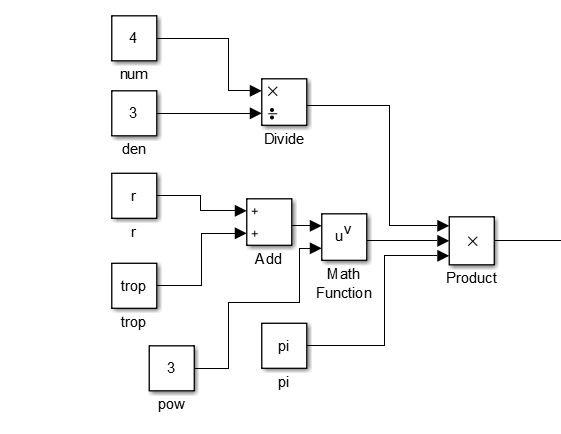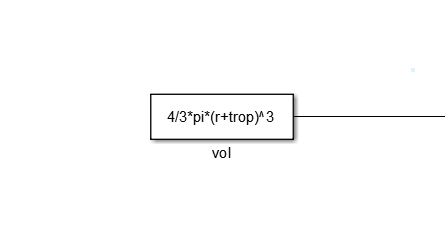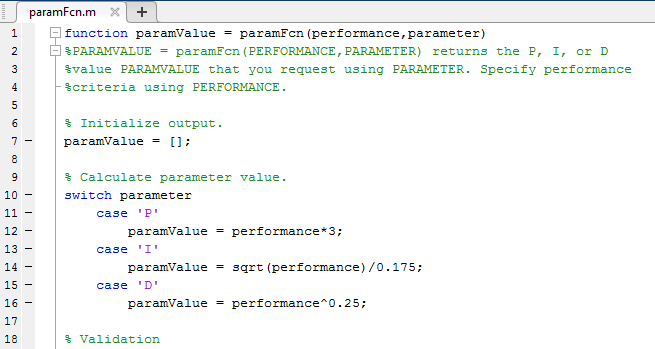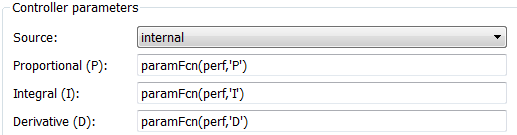• 如果使用变量和参数对象，则可以对变量和对象所代表的真实数量之间的代数关系进行显式建模。按照使用数学表达式设置变量值中所述在参数对象中使用表达式。

• 在编辑模块参数值中的表达式时，要导航到有关某函数的文档，请使用参数值旁边的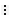按钮。您还可以导航到自定义函数的源代码。